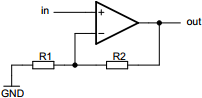# Non-inverting operational amplifier calculator

## Calculates properties of inverting operational amplifier circuit

 Example 1: Must calculate the resistance of R2 to have output of 70 millivolts at 800 microvolt input and R1 value of 10 kilo-ohms View example
 Input voltage volts Output voltage volts Resistor R1 ohms Resistor R2 ohmsYou may use one of the following SI prefix after a value: p=pico, n=nano, u=micro, m=milli, k=kilo, M=mega, G=giga

Fill in all values except the one you wish to calculate
• Input voltage - Input voltage to op-amp
• Output voltage - Output voltage from op-amp
• Resistor R1 - Resistance of resistor R1
• Resistor R2 - Resistance of resistor R2